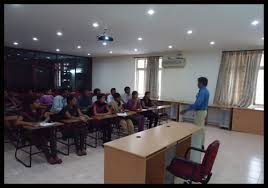## Low Memory Color Image Zero Tree Coding

Published on Jan 03, 2023

### Abstract

This paper presents a zero tree coding method for color images that uses no lists during encoding and decoding,permitting the omission of the lists requirement in Said and Pearlman's Set Partitioning In Hierarchical Trees (SPIHT) algorithm . Without the lists, the memory requirement in a VLSI implementation is reduced significantly.

This coding algorithm is also developed to reduce the circuit complexity of an implementation. Our experimental results show only a minor reduction of PSNR values when compared with the PSNR values obtained by the SPIHT codec illustrating well the trade-off between memory requirement and hardware simplicity.

### Introduction

Since being introduced by Shapiro , the zerotree wavelet image coding has been a well-recognized image coding method and based on the zerotree theory several coding algorithms have be developed. SPIHT is the most significant algorithm because it demonstrates a very sim-pleand efficient way to code a discrete wavelet transformed (DWT) image.

However, a SPIHT codec needs to main-tain three lists during coding and decoding to store the co-ordinates of significance coefficients and subset trees in the sorting order. The three lists become drawbacks for a hard-ware implementation because a large amount of memory is needed to maintain these lists. For color image coding the memory demand increases significantly.

For example, for a 512x512 color image, one single entry of the list needs 18 bits of memory to store the row and column coordinates. Given that the total number of list entries of a single color element is approximately twice the total number of coeffi-cients,the total memory required is 3.375 MBytes 1 and the required memory will increase if the bit rate increases.This 118(bits)x512(pixels)x512(lines)x3(colors)x2/8bits/1K/1K = 3.375MB high memory requirement makes SPIHT not a cost effective compression algorithm for VLSI implementation.

In this paper we present a zerotree coding algorithm for color image coding called Listless Zerotree Coding (LZC). The advantage of LZC over SPIHT is that no lists are needed during coding and decoding. Instead, a color co-efficient only needs a 3-bit flag if the coefficient is in the first wavelet transform level and a 6-bit flag if it is in any other transform level. Consequently, the amount of memory that required by a LZC codec is only a fraction of the amount needed by a SPIHT codec. In common with SPIHT, LZC is a progressive coding algorithm.

The color compo-nents are coded in the sequence of Y tree, V tree, then U tree, and the coding can stop at any point to give a precise bit-rate control.
LZC coding algorithm and SPIHT are quite alike. How-ever, since the usage of lists had been abandoned by LZC, different tree structure and coding procedure were developed for LZC.

The tree symbols of LZC zero tree are ex-plained as follow.

_ C(i,j) wavelet coefficient at the coordinate (i,j);

_ O(i,j) set of child coefficients of C(i,j), ie. Coefficients at coordinates (2i,2j), (2i,2j+1), (2i+1,2j), (2i+1,2j+1); except at the finest transform level (ie. Level 1);

_ D(i,j) set of descendant coefficients of C(i,j), ie. all offsprings of C(i,j);

_ F C (i,j) significant map of coefficient C(i,j);

_ F D (i,j) significant map of set D(i,j);

_ R(i,j) set of root coefficients at LL band.

_ LZC's zerotree relations adopt Shapiro's zerotree relation .

The positions of significant pixels are encoded by symbol Cand symbol D. The maps used to indicate the significance of C C and D D (ie. storing temporary zerotree structure) arefd map and fcfC map, re-spectively, as shown in Figure 1(b).

The size of Fc map is same size as the image. Whereas the size of FD map is only a quarter of the image because coefficients in level 1 do not have any descendants. Therefore, for a 512x512 color image, the total memory required to store zerotree structure is only 120KBytes (2) for all bit rates. Comparing to the 3.375 MBytes memory requirement for SPIHT, memory requirement for LZC has been reduced significantly.

 Are you interested in this topic.Then mail to us immediately to get the full report. email :- contactv2@gmail.com